Related Articles
How to select rows from a dataframe based on column values ?
• Last Updated : 01 Oct, 2020

Prerequisite: Pandas.Dataframes in Python

The rows of a dataframe can be selected based on conditions as we do use the SQL queries. The various methods to achieve this is explained in this article with examples. To explain the method a dataset has been created which contains data of points scored by 10 people in various games. The dataset is loaded into the dataframe and visualized first. Ten people with unique player id(Pid) have played different games with different game id(game_id) and the points scored in each game is added as an entry to the table. Some of the player’s points are not recorded and thus NaN value appears in the table.

Note: To get the CSV file used, click here.

## Python3

 `import` `pandas as pd`` ` `df``=``pd.read_csv(r``"__your file path__\example2.csv"``)``print``(df)`

Output: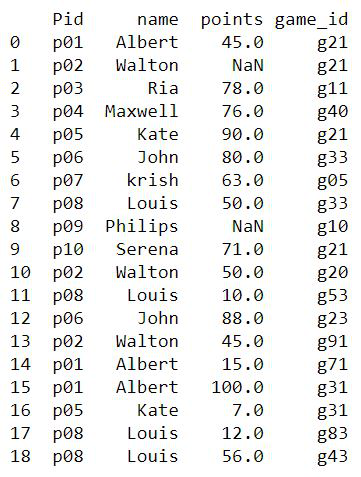dataset example2.csv

## Boolean Indexing method

In this method, for a specified column condition, each row is checked for true/false. The rows which yield True will be considered for the output. This can be achieved in various ways. The query used is Select rows where the column Pid=’p01′

Example 1: Checking condition while indexing

## Python3

 `# Choose entries with id p01``df_new ``=` `df[df[``'Pid'``] ``=``=` `'p01'``]`` ` `print``(df_new)`

Output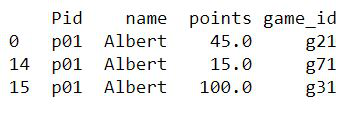Example 2: Specifying the condition ‘mask’ variable

The selected rows are assigned to a new dataframe with the index of rows from old dataframe as an index in the new one and the columns remaining the same.

## Python3

 `# condition mask``mask ``=` `df[``'Pid'``] ``=``=` `'p01'`` ` `# new dataframe with selected rows``df_new ``=` `pd.DataFrame(df[mask])`` ` `print``(df_new)`

Output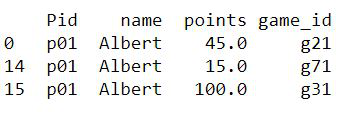Example 3: Combining mask and dataframes.values property

The query here is Select the rows with game_id ‘g21’.

## Python3

 `# condition with df.values property``mask ``=` `df[``'game_id'``].values ``=``=` `'g21'`` ` `# new dataframe``df_new ``=` `df[mask]`` ` `print``(df_new)`

Output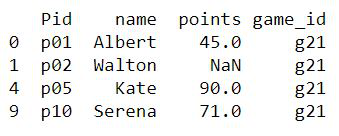## Positional indexing

The methods loc() and iloc() can be used for slicing the dataframes in Python. Among the differences between loc() and iloc(), the important thing to be noted is iloc() takes only integer indices, while loc() can take up boolean indices also.

Example 1: Using loc()

The mask gives the boolean value as an index for each row and whichever rows evaluate to true will appear in the result. Here, the query is to select the rows where game_id is g21.

## Python3

 `# for boolean indexing``mask ``=` `df[``'game_id'``].values ``=``=` `'g21'`` ` `# using loc() method``df_new ``=` `df.loc[mask]`` ` `print``(df_new)`

OutputExample 2: Using iloc()

The query is the same as the one taken above. The iloc() takes only integers as an argument and thus, the mask array is passed as a parameter to the numpy’s flatnonzero() function that returns the index in the list where the value is not zero (false)

## Python3

 `# condition mask``mask ``=` `df[``'game_id'``].values ``=``=` `'g21'``print``(``"Mask array :"``, mask)`` ` `# getting non zero indices``pos ``=` `np.flatnonzero(mask)``print``(``"\nRows selected :"``, pos)`` ` `# selecting rows``df.iloc[pos]`

Output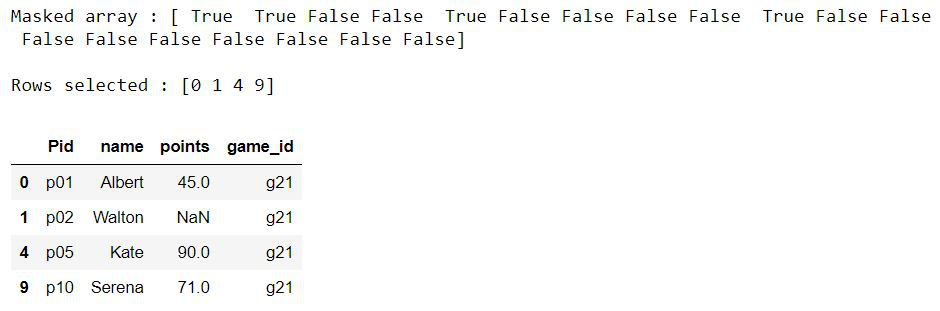## Using dataframe.query()

The query() method takes up the expression that returns a boolean value, processes all the rows in the dataframe, and returns the resultant dataframe with selected rows.

Example 1: Select  rows where name=”Albert”

## Python3

 `df.query(``'name=="Albert"'``)`

Output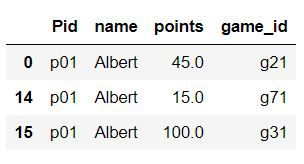Example 2: Select rows where points>50 and the player is not Albert.

This example is to demonstrate that logical operators like AND/OR can be used to check multiple conditions.

## Python3

 `df.query(``'points>50 & name!="Albert"'``)`

Output## Using isin()

This method of dataframe takes up an iterable or a series or another dataframe as a parameter and checks whether elements of the dataframe exists in it. The rows whichever evaluates to true are considered for the resultant.

Example 1: Select the rows where players are Albert, Louis, and John.

## Python3

 `#Players to be selected``li``=``[``'Albert'``,``'Louis'``,``'John'``]`` ` `df[df.name.isin(li)]`

Output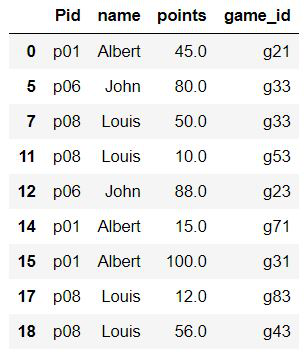Example 2: Select rows where points>50 and players are not Albert, Louis and John.

The tiled symbol (~) provides the negation of the expression evaluated.

## Python3

 `# values to be present in selected rows``li ``=` `[``'Albert'``, ``'Louis'``, ``'John'``]`` ` `# selecting rows from dataframe``df[(df.points > ``50``) & (~df.name.isin(li))]`

Output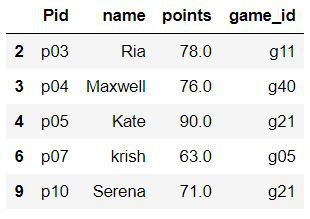## Using np.where()

The numpy’s where() function can be combined with the pandas’ isin() function to produce a faster result. The numpy.where() is proved to produce results faster than the normal methods used above.

Example:

## Python3

 `import` `numpy as np`` ` `df_new ``=` `df.iloc[np.where(df.name.isin(li))]`

Output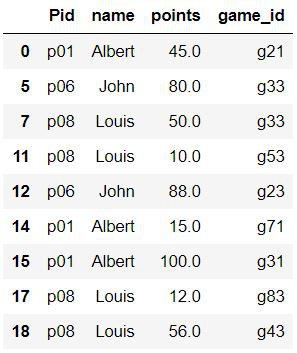## Python3

 `# to calculate timing``import` `numpy as np``%` `%` `timeit`` ` ` ` `# using mixture of numpy and pandas method``df_new ``=` `df.iloc[np.where(df.name.isin(li))]`

Output:

756 µs ± 132 µs per loop (mean ± std. dev. of 7 runs, 1000 loops each)

## Python3

 `# to calculate time``%``%``timeit`` ` `li``=``[``'Albert'``,``'Louis'``,``'John'``]`` ` `# Pandas method only``df[(df.points>``50``)&(~df.name.isin(li))]`

Output

1.7 ms ± 307 µs per loop (mean ± std. dev. of 7 runs, 1000 loops each)

Attention geek! Strengthen your foundations with the Python Programming Foundation Course and learn the basics.

To begin with, your interview preparations Enhance your Data Structures concepts with the Python DS Course.

My Personal Notes arrow_drop_up